# GRAPH THEORY: Let G be a graph whose vertex set is a set V = {p1, p2, . . . , p6 } of six people....

GRAPH THEORY:

Let G be a graph whose vertex set is a set V = {p1, p2, . . . , p6 } of six people. PROVE that there exist three people who are all friends with each other, or three people none of whom are friends with each other.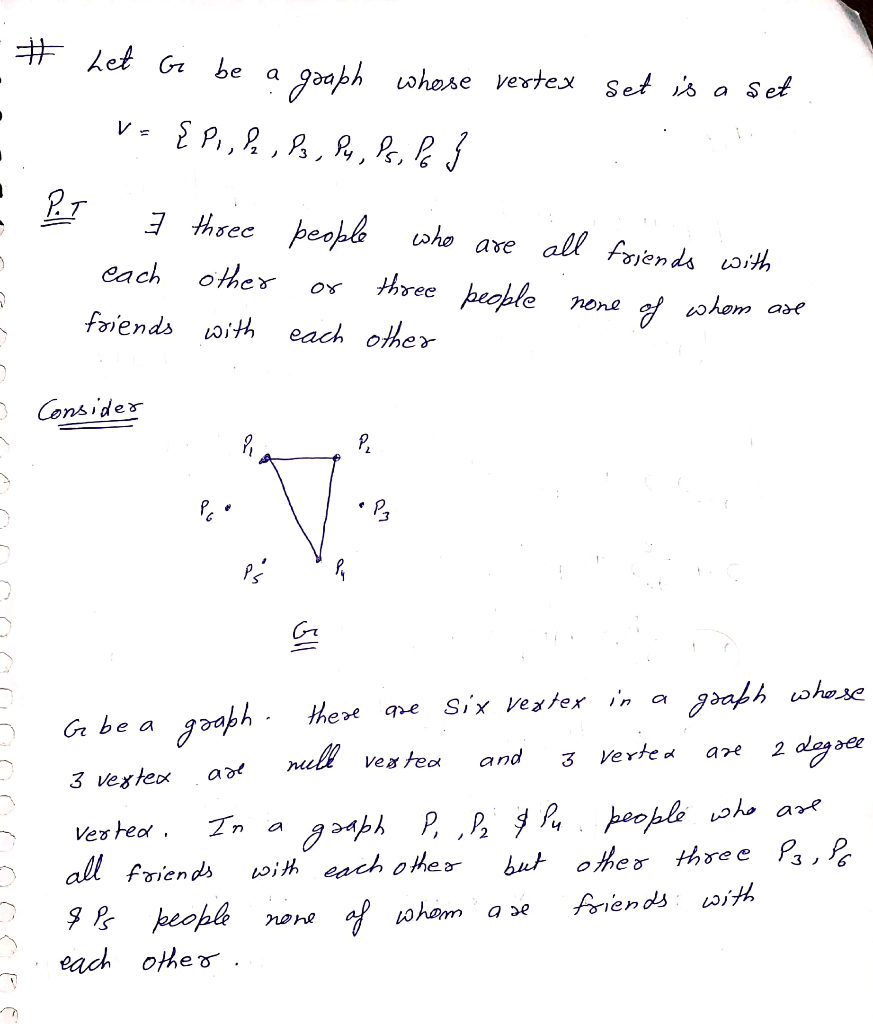##### Add Answer of: GRAPH THEORY: Let G be a graph whose vertex set is a set V = {p1, p2, . . . , p6 } of six people....
Similar Homework Help Questions
• ### Question 4. For n 2, let Gn be the grid graph, whose vertex set is V={(x, y) E Z × Z : 0 < x < n,0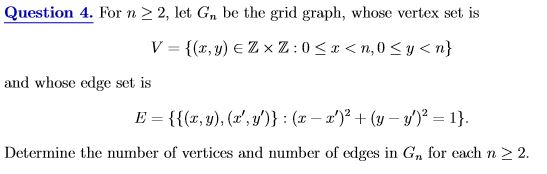topic: graph theory Question 4. For n 2, let Gn be the grid graph, whose vertex set is V={(x, y) E Z × Z : 0 < x < n,0

• ### Let G = (V;E) be an undirected and unweighted graph. Let S be a subset of the vertices. The graph...

Let G = (V;E) be an undirected and unweighted graph. Let S be a subset of the vertices. The graph induced on S, denoted G[S] is a graph that has vertex set S and an edge between two vertices u, v that is an element of S provided that {u,v} is an edge of G. A subset K of V is called a killer set of G if the deletion of K kills all the edges of G, that is...

• ### Let G -(V, E) be a graph. The complementary graph G of G has vertex set V. Two vertices are adjacent in G if and only if they are not adjacent in G. (a) For each of the following graphs, describe its...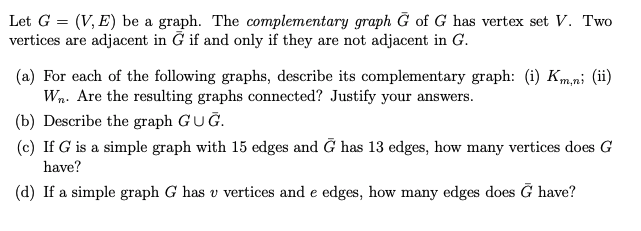Let G -(V, E) be a graph. The complementary graph G of G has vertex set V. Two vertices are adjacent in G if and only if they are not adjacent in G. (a) For each of the following graphs, describe its complementary graph: (i) Km,.ni (i) W Are the resulting graphs connected? Justify your answers. (b) Describe the graph GUG. (c) If G is a simple graph with 15 edges and G has 13 edges, how many vertices does...

• ### introduction to graph theory (23)23.(Prove Theorem 11.1.3) Let G = (V, E) be ageneral graph. For each vertex x, letC(x) = {z: x is equivalent to z}.i)for all vetices xand y, either C(x)= C(y) or else .In other words ofthe setsC(x) and C(y) cannot intersect unless they are equal.ii)In . Then there does not exist an edgejoining a vertex inC(x)to a vertex in C(y).Theorem 11.1.3 Let G= (V, E) be a generalgraph.Then the vertexset V can be uniquelypartitioned intononemptypartsV1,V2,V3,...,Vk, sothat the following conditions are...

• ### read exercise and do question 6 In problems 3 through 6, imagine putting a small cube inside a larger cube and let G be the graph whose vertex set consists of the 8 corners of the small cube an...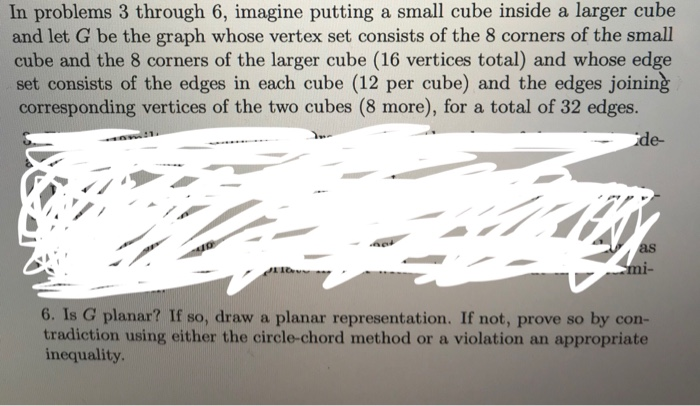read exercise and do question 6 In problems 3 through 6, imagine putting a small cube inside a larger cube and let G be the graph whose vertex set consists of the 8 corners of the small cube and the 8 corners of the larger cube (16 vertices total) and whose edge set consists of the edges in each cube (12 per cube) and the edges joining corresponding vertices of the two cubes (8 more), for a total of 32...

• ### Let n >k> 1 with n even and k odd. Make a k-regular graph G by putting n vertices in a circle and...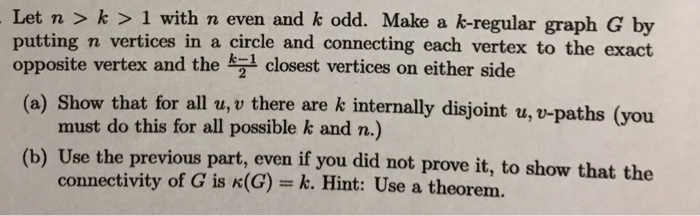need help with a and b in this graph theory question Let n >k> 1 with n even and k odd. Make a k-regular graph G by putting n vertices in a circle and connecting each vertex to the exact a) Show that for all u,v there are k internally disjoint u, v-paths (you (b) Use the previous part, even if you did not prove it, to show that the e vertex and the k 1 closest vertices on either...

• ### answer question 3 , imagine putting a small cube inside a larger cube and let G be the graph whose vertex set consists of the 8 corners of the small cube and the 8 corners of the larger cube (1...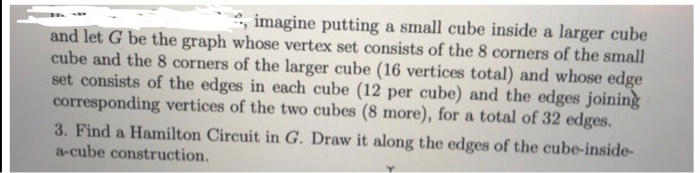answer question 3 , imagine putting a small cube inside a larger cube and let G be the graph whose vertex set consists of the 8 corners of the small cube and the 8 corners of the larger cube (16 vertices total) and whose edge set consists of the edges in each cube (12 per cube) and the edges joinin corresponding vertices of the two cubes (8 more), for a total of 32 edges. 3. Find a Hamilton Circuit in...

• ### Ems B-1 Graph coloring Given an undirected graph G (V. E), a k-coloring of G is a function c : V ...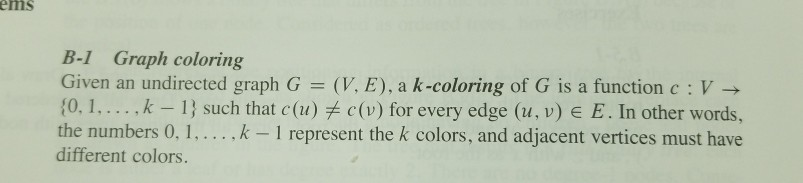ems B-1 Graph coloring Given an undirected graph G (V. E), a k-coloring of G is a function c : V - (0. I. . . . .k-1) such that c (11)关c (v) for every edge (u, v) E E. In other words, the numbers 0.1,... k 1 represent the k colors, and adjacent vertices different colors. must have c. Let d be the maximum degree of any vertex in a graph G. Prove that we can color G with...

• ### Let G (V, E) be a directed graph with n vertices and m edges. It is known that in dfsTrace of G t...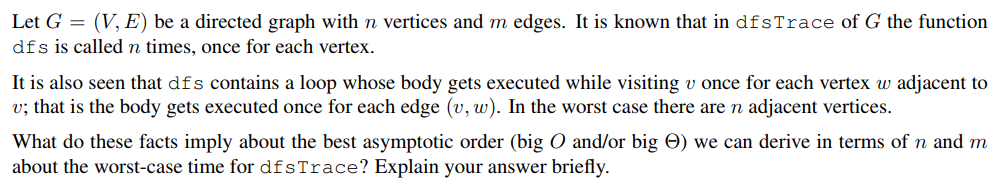Let G (V, E) be a directed graph with n vertices and m edges. It is known that in dfsTrace of G the function dfs is called n times, once for each vertex It is also seen that dfs contains a loop whose body gets executed while visiting v once for each vertex w adjacent to v; that is the body gets executed once for each edge (v, w). In the worst case there are n adjacent vertices. What do...

• ### ) A vartex cover is n set af vertices for which esch edge has at lesst ane of its vertices in the set. What is the size of the smallest vertex ㏄ver in the Petersen graph? Give an example of such a s...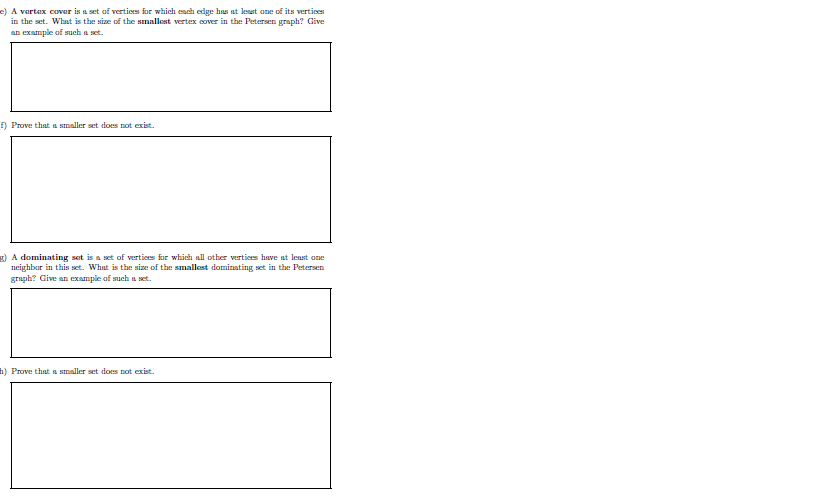) A vartex cover is n set af vertices for which esch edge has at lesst ane of its vertices in the set. What is the size of the smallest vertex ㏄ver in the Petersen graph? Give an example of such a set Prove that a smaller set does not exist. A dominating sot is a set of vertices for which all other vertices have nt lenst ane neighbar in this set. What is the e of the smallest dominating...

Need Online Homework Help?